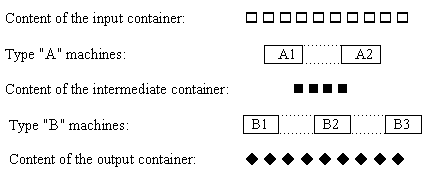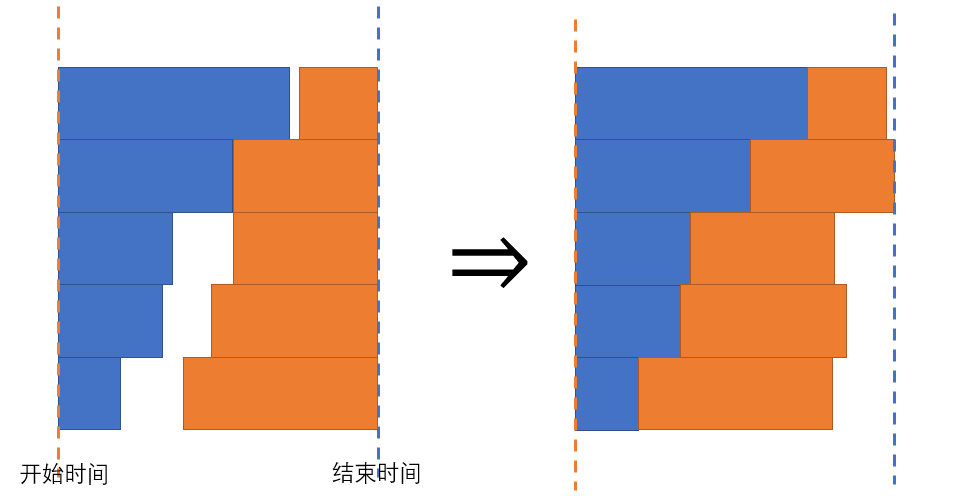# 洛谷 P2751 [USACO4.2]工序安排Job Processing 题解【贪心】

类似排队接水？

## 题目描述5 2 3
1 1 3 1 4

3 5

## 说明

USACO Training Section 4.2

## 题解：

第一问，类似排队接水，只不过贪心的条件变了。设si为队列i的原长，mi为队列i每接一次水耗费的时间。因为每个队伍的mi不同，所以我们要找si+mi最小的队列，排在它后面，更新si长度为si+mi。因为放置工件不分先后顺序，所以在队列里也是可以前后互换的，就如DP中的无后效性，第一问就做出来了。

第二问，假设与第一问无关，我们认为当前时间点上，所有工件都可以使用，那么问题又演变成了同样的排队接水问题。因为同一工件的加工时间不能重叠，同一机器的加工时间也不能重叠，同时保证加工时间最长的工件时间最小（有点像二分？）。而前后加工的工件如果不重合，是可以任意匹配的，所以我们把第一个机器时间第i长的与第二个机器时间倒数第i长的配对，把它们当作一个工件。假设所有工件一起开始，一起结束，找到所有配对中和最大的当作开始时间与结束时间的间隔，就会使所有工件不自相重合。并且第一个机器最长的配了第二个机器最小的，根据名次推下去，总匹配到最优的工序。

如图，在左边找到了最优匹配方案（保证了最长的最小），我们如果将它对齐，会得到右边的图，因为一定有至少一条位置不会改变，所以也证明了它是最长加工时间的工件。以后遇到这种进程题目最好往平移的方面去想，最后再想办法把它拼起来，虽然不一定所有的都符合，但是记住这个思路，就多一种方法。例如[HNOI2001]产品加工 题解

## Code：

#include<cstdio>
#include<cstring>
int a,b;
int m1,m2;
int s1,s2;
int main()
{
int n,ans=0;
scanf("%d%d%d",&n,&m1,&m2);
m1=0x3fffffff;
m2=0x3fffffff;
for(int i=1;i<=m1;i++)
scanf("%d",&m1[i]);
for(int i=1;i<=m2;i++)
scanf("%d",&m2[i]);
for(int i=1;i<=n;i++)//第一遍贪心
{
int tmp=31;
for(int j=1;j<=m1;j++)
if(a[j]+m1[j]<a[tmp]+m1[tmp])
tmp=j;
a[tmp]+=m1[tmp];
s1[i]=a[tmp];
ans=ans>s1[i]?ans:s1[i];
}
printf("%d ",ans);//第一问
for(int i=1;i<=n;i++)//第二遍贪心
{
int tmp=31;
for(int j=1;j<=m2;j++)
if(b[j]+m2[j]<b[tmp]+m2[tmp])
tmp=j;
b[tmp]+=m2[tmp];
s2[i]=b[tmp];
}
ans=0;
for(int i=1;i<=n;i++)//拼接两问答案
ans=ans>s1[i]+s2[n-i+1]?ans:s1[i]+s2[n-i+1];
printf("%d\n",ans);
return 0;
}


### 说点什么Subscribe

/* */